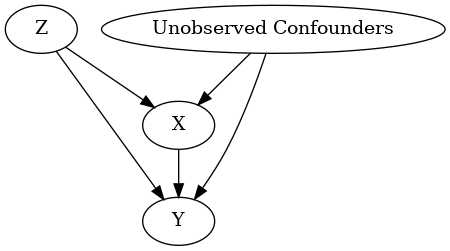# Different ways to load an input graph

We recommend using the GML graph format to load a graph. You can also use the DOT format, which requires additional dependencies (either pydot or pygraphviz).

DoWhy supports both loading a graph as a string, or as a file (with the extensions ‘gml’ or ‘dot’).

Below is an example showing the different ways of loading the same graph.

:

import os, sys
import random
sys.path.append(os.path.abspath("../../../"))

:

import numpy as np
import pandas as pd

import dowhy
from dowhy import CausalModel
from IPython.display import Image, display


## I. Generating dummy data

We generate some dummy data for three variables: X, Y and Z.

:

z=[i for i in range(10)]
random.shuffle(z)
df = pd.DataFrame(data = {'Z': z, 'X': range(0,10), 'Y': range(0,100,10)})
df

:

Z X Y
0 0 0 0
1 7 1 10
2 8 2 20
3 6 3 30
4 5 4 40
5 3 5 50
6 9 6 60
7 2 7 70
8 1 8 80
9 4 9 90

### GML format

:

# With GML string
model=CausalModel(
data = df,
treatment='X',
outcome='Y',
graph="""graph[directed 1 node[id "Z" label "Z"]
node[id "X" label "X"]
node[id "Y" label "Y"]
edge[source "Z" target "X"]
edge[source "Z" target "Y"]
edge[source "X" target "Y"]]"""

)
model.view_model()

display(Image(filename="causal_model.png"))

INFO:dowhy.causal_graph:If this is observed data (not from a randomized experiment), there might always be missing confounders. Adding a node named "Unobserved Confounders" to reflect this.
INFO:dowhy.causal_model:Model to find the causal effect of treatment ['X'] on outcome ['Y']:

# With GML file
model=CausalModel(
data = df,
treatment='X',
outcome='Y',
graph="../example_graphs/simple_graph_example.gml"
)
model.view_model()

display(Image(filename="causal_model.png"))

INFO:dowhy.causal_graph:If this is observed data (not from a randomized experiment), there might always be missing confounders. Adding a node named "Unobserved Confounders" to reflect this.
INFO:dowhy.causal_model:Model to find the causal effect of treatment ['X'] on outcome ['Y']### DOT format

:

# With DOT string
model=CausalModel(
data = df,
treatment='X',
outcome='Y',
graph="digraph {Z -> X;Z -> Y;X -> Y;}"
)
model.view_model()

from IPython.display import Image, display
display(Image(filename="causal_model.png"))

INFO:dowhy.causal_graph:If this is observed data (not from a randomized experiment), there might always be missing confounders. Adding a node named "Unobserved Confounders" to reflect this.
INFO:dowhy.causal_model:Model to find the causal effect of treatment ['X'] on outcome ['Y']:

# With DOT file
model=CausalModel(
data = df,
treatment='X',
outcome='Y',
graph="../example_graphs/simple_graph_example.dot"
)
model.view_model()

display(Image(filename="causal_model.png"))

INFO:dowhy.causal_graph:If this is observed data (not from a randomized experiment), there might always be missing confounders. Adding a node named "Unobserved Confounders" to reflect this.
INFO:dowhy.causal_model:Model to find the causal effect of treatment ['X'] on outcome ['Y']